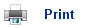# Energy and Entropy

• Substances can either be solids, liquids or gases at a given temperature.
• The particles in solids are in a fixed position within a lattice; this is why solids are rigid.
• The particles in liquids and gases are free to move around; the substances are fluids and can take up any shape.
• On heating, solids and liquids do not expand; however gases expand significantly.
• Gases can also be compressed, whereas solids and liquids cannot. The ideal gas equation shows how pressure (p), volume (V) and temperature (T) are all related for a fixed amount of gas (n):

pV = nRT

• On heating a solid, liquid or gas, its temperature increases; except for during the transition points between a solid and liquid (when it melts) and a liquid and gas (when it boils).

## Energy

• The specific heat capacity (cp) is a measure of how easy it is to warm something up.
• The specific heat capacity tells us how many joules of energy are required to raise the temperature of 1g of a substance by 1K (units are J g-1 K-1).
• The temperature of a substance is related to the kinetic energy of its particles; a substance feels hotter if the particles are moving more energetically.
• There are three forms of kinetic energy:
1. Translation- moving of the whole particle from one place to another.
2. Rotation- spinning around.
3. Vibration- stretching and compressing of the bond lengths and bond angles.
• At greater energies, electronic changes or even bond breaking can occur; however these changes do not affect the temperature as they do not affect the motion of the particles.
• Energy is quantised; the chemical particles are restricted to particular levels of electronic, vibrational, rotational and translational energy.
• The gaps between the energy levels increase in the order:

Translational < Rotational < Vibrational < Electronic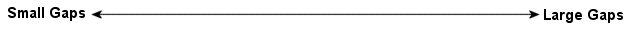• For example, a simple molecule, such as H2, may have the following energy levels: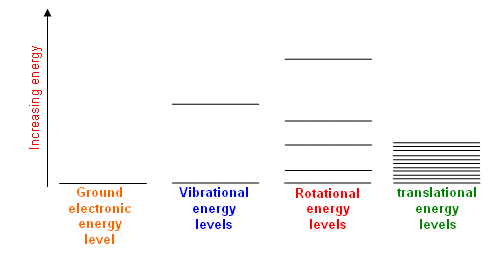• Each type of electronic level has its own stack of energy values.
• So, overall, there are a large number of closely spaced energy levels for the particles to be in; there are even more for complicated molecules.
• However; the particles do not all have the same energy; their energy values are spread out.
• Their energies are constantly changing too; they are gaining and losing energy as they collide and exchange energy.
• The particles are distributed amongst the energy values that give them the greatest entropy value.

## Entropy

• Entropy is a measure of the disorder of a system. It can also be expressed as a measure of the number of ways something can be arranged.
• Mixing and diffusion occur due to the increase in entropy that occurs when the particles become more spread out.
• Similarly, there are more ways of arranging the energies of the particles if they are spread out in an energy level stack.
• This can be shown in the following example:
• Imagine that two particles have four quanta (a “packet” of energy) between them. If they both had two quanta each, they could only be arranged in one way: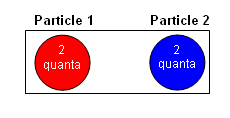• However, if the quanta were spread out amongst the energy stacks, there would be five possible arrangements of the particles: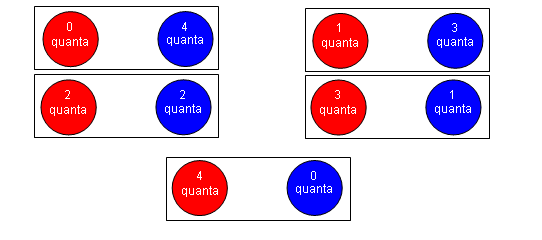• The particles are made hotter by giving them six quanta of energy: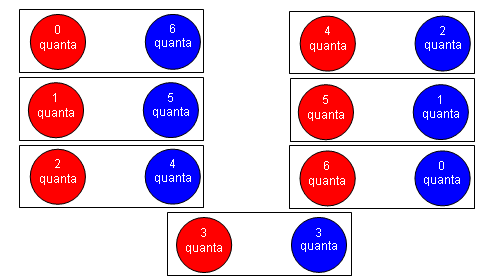• With more energy, there are more possible arrangements of the quanta; therefore the entropy is greater.
• The entropy also increases if the number of particles sharing the energy is increased; for example, there are fifteen ways in which three particles can share 4 quanta.
• The number of quanta can also be increased without increasing the temperature if there are more energy levels that are not as closely spread; the quanta needed to change the energy levels are therefore smaller, so there must be more of them for the same total energy.
• With a lot of particles (6 x 1023 for example), sharing a lot of quanta, the higher entropy associated with the spread of energies makes the distribution of energies inevitable.
• Generally, solids have lower entropies than liquids, which in turn have lower entropies than gases.
• This is due to the fact that solids have a regular lattice structure, whereas the particles in liquids and gases are arranged randomly. Gases have a higher entropy than liquids, because they occupy a greater volume than liquids and so have more positions in which they can be arranged.
• The energy levels tend to be more closely spaced for molecules which contain heavier atoms.
• The number of energy levels tends to increase with the number of atoms in a molecule, thus making adjacent energy levels closer together in larger molecules (in order to spread the quanta).
• Both these factors lead to higher entropy values.

## Summary

• Entropy is a measure of the number of ways of arranging particles and their quanta of energy.
• A collection of particles has a greater entropy if they are spread out by as much as possible.
• There are more ways of arranging the energy of a collection of particles if they are spread out among the energy levels available to them. The more complicated the molecule, the greater the number of energy levels and so the greater the entropy.
• The entropy is increased if the energy is shared between more particles.
• The entropy is increased if there are more quanta of energy; this happens when:
1. The energy levels are more closely spaced.
2. The temperature is higher.

## Useful books for revision

Revise A2 Chemistry for Salters (OCR A Level Chemistry B)Salters (OCR) Revise A2 ChemistryHome